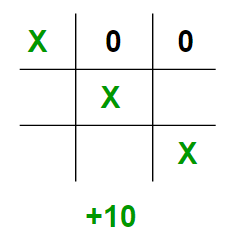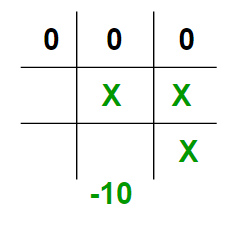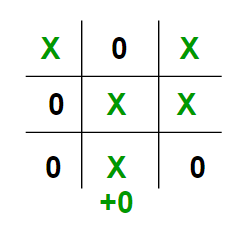Minimax Algorithm in Game Theory | Set 2 (Introduction to Evaluation Function)

• Difficulty Level : Easy
• Last Updated : 24 Aug, 2021

Prerequisite : Minimax Algorithm in Game Theory
As seen in the above article, each leaf node had a value associated with it. We had stored this value in an array. But in the real world when we are creating a program to play Tic-Tac-Toe, Chess, Backgamon, etc. we need to implement a function that calculates the value of the board depending on the placement of pieces on the board. This function is often known as Evaluation Function. It is sometimes also called Heuristic Function.
The evaluation function is unique for every type of game. In this post, evaluation function for the game Tic-Tac-Toe is discussed. The basic idea behind the evaluation function is to give a high value for a board if maximizer‘s turn or a low value for the board if minimizer‘s turn.
For this scenario let us consider X as the maximizer and O as the minimizer.
Let us build our evaluation function :

1. If X wins on the board we give it a positive value of +10.1. If O wins on the board we give it a negative value of -10.1. If no one has won or the game results in a draw then we give a value of +0.We could have chosen any positive / negative value other than 10. For the sake of simplicity we chose 10 for the sake of simplicity we shall use lower case ‘x’ and lower case ‘o’ to represent the players and an underscore ‘_’ to represent a blank space on the board.
If we represent our board as a 3×3 2D character matrix, like char board; then we have to check each row, each column and the diagonals to check if either of the players have gotten 3 in a row.

C++

 // C++ program to compute evaluation function for// Tic Tac Toe Game.#include#includeusing namespace std; // Returns a value based on who is winning// b is the Tic-Tac-Toe boardint evaluate(char b){    // Checking for Rows for X or O victory.    for (int row = 0; row<3; row++)    {        if (b[row]==b[row] && b[row]==b[row])        {            if (b[row]=='x')               return +10;            else if (b[row]=='o')               return -10;        }    }     // Checking for Columns for X or O victory.    for (int col = 0; col<3; col++)    {        if (b[col]==b[col] && b[col]==b[col])        {            if (b[col]=='x')                return +10;            else if (b[col]=='o')                return -10;        }    }     // Checking for Diagonals for X or O victory.    if (b==b && b==b)    {        if (b=='x')            return +10;        else if (b=='o')            return -10;    }    if (b==b && b==b)    {        if (b=='x')            return +10;        else if (b=='o')            return -10;    }     // Else if none of them have won then return 0    return 0;} // Driver codeint main(){    char board =    {        { 'x', '_', 'o'},        { '_', 'x', 'o'},        { '_', '_', 'x'}    };     int value = evaluate(board);    printf("The value of this board is %d\n", value);    return 0;}

Java

 // Java program to compute evaluation function for// Tic Tac Toe Game. class GFG{ // Returns a value based on who is winning// b is the Tic-Tac-Toe boardstatic int evaluate(char b[][]){    // Checking for Rows for X or O victory.    for (int row = 0; row < 3; row++)    {        if (b[row] == b[row] && b[row] == b[row])        {            if (b[row] == 'x')            return +10;            else if (b[row] == 'o')            return -10;        }    }     // Checking for Columns for X or O victory.    for (int col = 0; col < 3; col++)    {        if (b[col] == b[col] && b[col] == b[col])        {            if (b[col] == 'x')                return +10;            else if (b[col] == 'o')                return -10;        }    }     // Checking for Diagonals for X or O victory.    if (b == b && b == b)    {        if (b == 'x')            return +10;        else if (b == 'o')            return -10;    }    if (b == b && b == b)    {        if (b == 'x')            return +10;        else if (b == 'o')            return -10;    }     // Else if none of them have won then return 0    return 0;} // Driver codepublic static void main(String[] args){    char board[][] =    {        { 'x', '_', 'o'},        { '_', 'x', 'o'},        { '_', '_', 'x'}    };     int value = evaluate(board);    System.out.printf("The value of this board is %d\n", value);}} // This code is contributed by PrinciRaj1992

Python3

 # Python3 program to compute evaluation# function for Tic Tac Toe Game. # Returns a value based on who is winning# b is the Tic-Tac-Toe boarddef evaluate(b):      # Checking for Rows for X or O victory.    for row in range(0, 3):              if b[row] == b[row] and b[row] == b[row]:                      if b[row] == 'x':                return 10            elif b[row] == 'o':                return -10     # Checking for Columns for X or O victory.    for col in range(0, 3):              if b[col] == b[col] and b[col] == b[col]:                      if b[col]=='x':                return 10            elif b[col] == 'o':                return -10     # Checking for Diagonals for X or O victory.    if b == b and b == b:              if b == 'x':            return 10        elif b == 'o':            return -10          if b == b and b == b:              if b == 'x':            return 10        elif b == 'o':            return -10          # Else if none of them have won then return 0    return 0  # Driver codeif __name__ == "__main__":      board = [['x', '_', 'o'],             ['_', 'x', 'o'],             ['_', '_', 'x']]          value = evaluate(board)    print("The value of this board is", value) # This code is contributed by Rituraj Jain

C#

 // C# program to compute evaluation function for// Tic Tac Toe Game.using System; class GFG{ // Returns a value based on who is winning// b[3,3] is the Tic-Tac-Toe boardstatic int evaluate(char [,]b){    // Checking for Rows for X or O victory.    for (int row = 0; row < 3; row++)    {        if (b[row, 0] == b[row, 1] && b[row, 1] == b[row, 2])        {            if (b[row, 0] == 'x')            return +10;            else if (b[row, 0] == 'o')            return -10;        }    }     // Checking for Columns for X or O victory.    for (int col = 0; col < 3; col++)    {        if (b[0, col] == b[1, col] && b[1, col] == b[2, col])        {            if (b[0, col] == 'x')                return +10;            else if (b[0, col] == 'o')                return -10;        }    }     // Checking for Diagonals for X or O victory.    if (b[0, 0] == b[1, 1] && b[1, 1] == b[2, 2])    {        if (b[0, 0] == 'x')            return +10;        else if (b[0, 0] == 'o')            return -10;    }    if (b[0, 2] == b[1, 1] && b[1, 1] == b[2, 0])    {        if (b[0, 2] == 'x')            return +10;        else if (b[0, 2] == 'o')            return -10;    }     // Else if none of them have won then return 0    return 0;} // Driver codepublic static void Main(String[] args){    char [,]board =    {        { 'x', '_', 'o'},        { '_', 'x', 'o'},        { '_', '_', 'x'}    };     int value = evaluate(board);    Console.Write("The value of this board is {0}\n", value);}} // This code is contributed by Rajput-Ji

Javascript



Output :

The value of this board is 10

The idea of this article is to understand how to write a simple evaluation function for the game Tic-Tac-Toe. In the next article we shall see how to combine this evaluation function with the minimax function. Stay Tuned.